Question

A proton is moving through a uniform potential difference of 300 V. If the proton enters the potential with a velocity of 1.80 * 10^5 m/s x, determine the velocity of the proton the moment it leaves the potential difference.

a) 2.40 * 10^5 m/s x

b) 3.00 * 10^5 m/s x

c) 8.99 *10^1 m/s x

d) There is not enough information to solve this problem.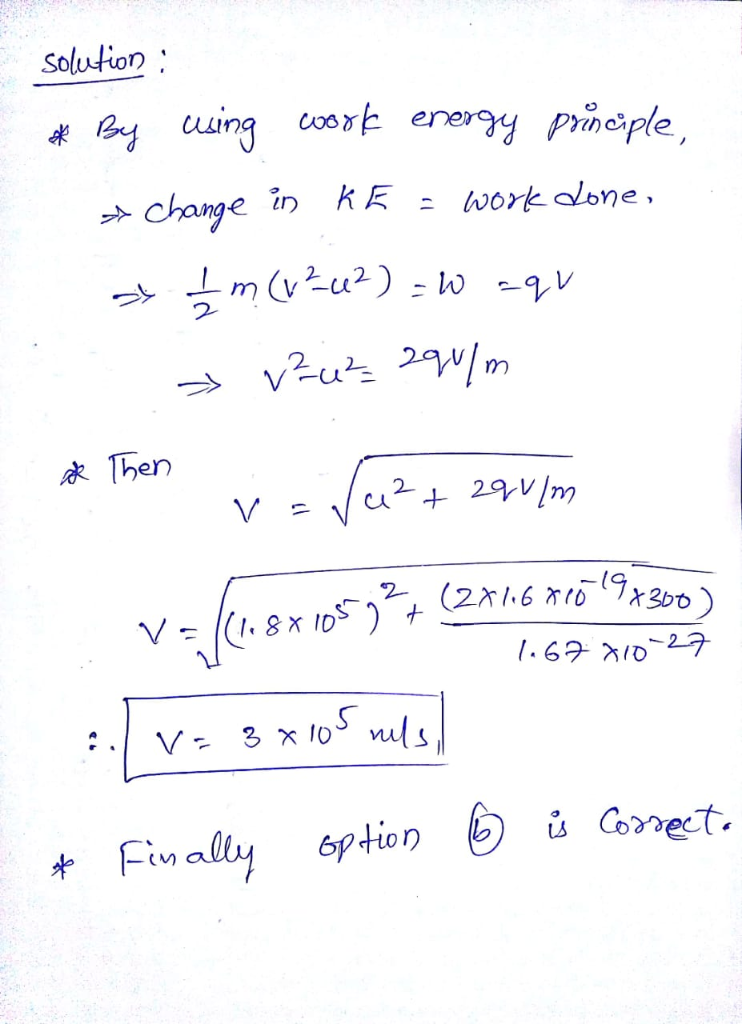#### Earn Coins

Coins can be redeemed for fabulous gifts.

Similar Homework Help Questions
• ### 2) A proton, initially moving at 6.00x105 m/s, goes through a potential difference of +1000.0 V...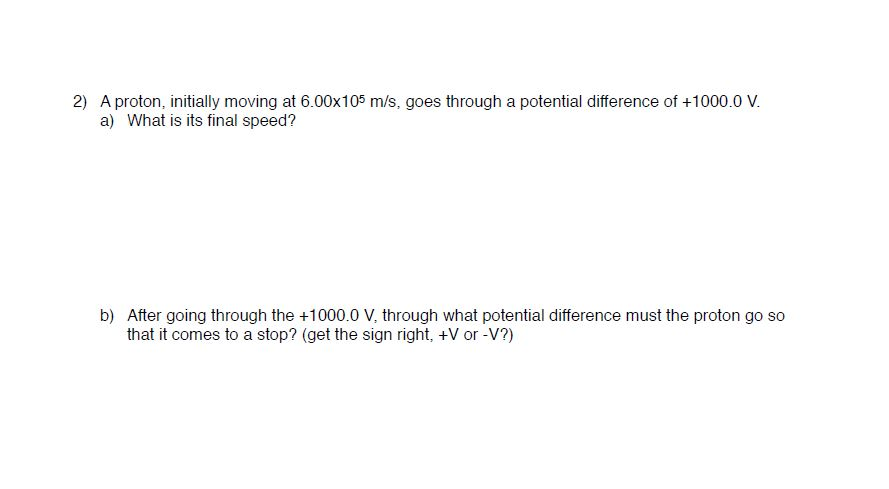2) A proton, initially moving at 6.00x105 m/s, goes through a potential difference of +1000.0 V a) What is its final speed? b) After going through the +1000.0 V, through what potential difference must the proton go so that it comes to a stop? (get the sign right, +V or -V?)

• ### Calculate the speed of an proton moving through a potential difference of 5.0 V. Repeat for...

Calculate the speed of an proton moving through a potential difference of 5.0 V. Repeat for an electron

• ### A proton traveling at v= 4.65 x 10° m/s to the right enters the region between...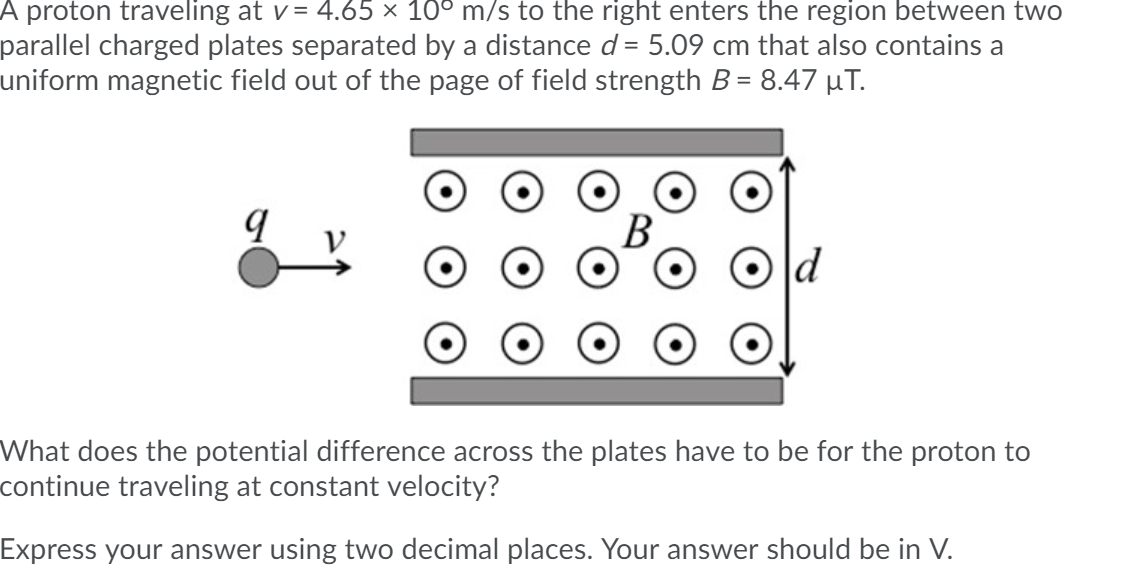A proton traveling at v= 4.65 x 10° m/s to the right enters the region between two parallel charged plates separated by a distance d = 5.09 cm that also contains a uniform magnetic field out of the page of field strength B = 8.47 ut. a What does the potential difference across the plates have to be for the proton to continue traveling at constant velocity? Express your answer using two decimal places. Your answer should be in V.

• ### An electron is accelerated from rest through a potential difference of 2600 V and then enters...

An electron is accelerated from rest through a potential difference of 2600 V and then enters a region where there is a uniform 1.40-T magnetic field. a) What is the magnitude of the magnetic force on the electron if it is moving in the direction of the magnetic field? b) What is the magnitude of the magnetic force on the electron if it is moving opposite to the direction of the magnetic field? c) What is the magnitude of the...

• ### A)A proton is accelerated from rest through a potential difference of 25707 V. What is the...

A)A proton is accelerated from rest through a potential difference of 25707 V. What is the kinetic energy of this proton after this acceleration? The mass of a proton is 1.673 × 10−27 kg and the elemental charge is 1.602 × 10−19 C. Answer in units of J. B) What is the speed of the proton after this acceleration? Answer in units of m/s.

• ### A proton of mass m = 1.67 x 10-27 kg and charge q = 1.60 x 10-19 C is accelerated from rest through a potential difference of 4000 V. What final velocity does it achieve? A proton of mass...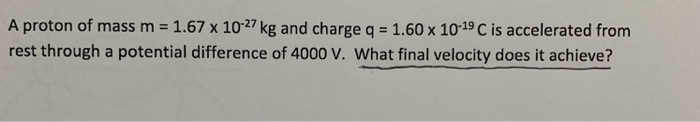A proton of mass m = 1.67 x 10-27 kg and charge q = 1.60 x 10-19 C is accelerated from rest through a potential difference of 4000 V. What final velocity does it achieve? A proton of mass m = 1.67 x 10-27 kg and charge q = 1.60 x 10-19 C is accelerated from rest through a potential difference of 4000 V. What final velocity does it achieve?

• ### A proton has be accelerated from rest through a uniform electric field. If the final velocity...

A proton has be accelerated from rest through a uniform electric field. If the final velocity of the proton is 5x10^5 m/s, what is the potential difference across the electric field?

• ### A proton is accelerated from rest through a potential differences of 1.0 kV. It enters a...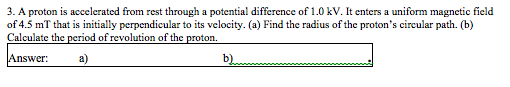A proton is accelerated from rest through a potential differences of 1.0 kV. It enters a uniform magnetic field of 4.5 mT that is initially perpendicular to its velocity. (a) Find the radius of the proton's circular path (b). Calculate the period of revolution of the proton.

• ### Question 8 (5 points) A proton moves through a region containing a uniform electric field given...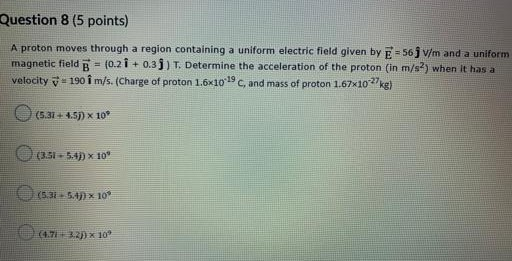Question 8 (5 points) A proton moves through a region containing a uniform electric field given by = 56 V/m and a uniform magnetic field B - (0.21 +0.3]) T. Determine the acceleration of the proton (in m/s) when it has a velocity v. 190 i m/s.(Charge of proton 1.6x10c, and mass of proton 1.67X10kg) (5.37+ 4.5)) x 10 (3.51 - 5:49 x 10 (5.32.5.47 x 10

• ### 2. An electron is accelerated from rest through a potential difference Δνι-800 V, and enters the...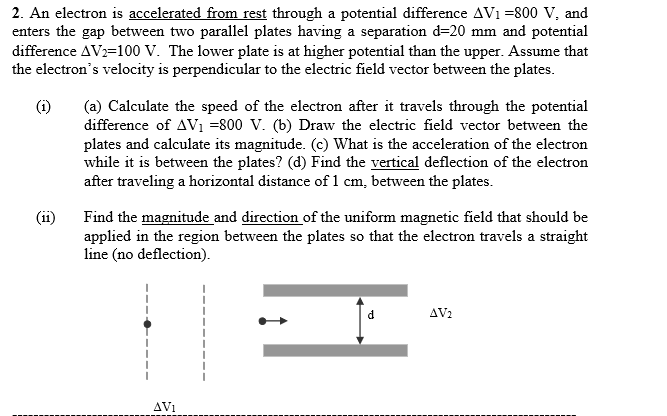2. An electron is accelerated from rest through a potential difference Δνι-800 V, and enters the gap between two parallel plates having a separation d-20 mm and potential difference AVF 100 V. The lower plate is at higher potential than the upper. Assume that the electron's velocity is perpendicular to the electric field vector between the plates (i) (a) Calculate the speed of the electron after it travels through the potential difference of A,-800 V. (b) Draw the electric field...